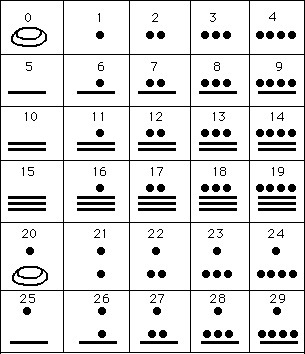Mayan Numeral System and Calendar – Sci-advent – Day 20The Mayas are one of greatest human civilisations. Not only did they have excellent agriculture, pottery and hieroglyph writing, but also have some of the most impressive architecture and symbolic art as well as mathematics, astronomy and calendar-making. It is said that they had predicted the “end of the world”, but I would like to think of it as the end and beginning of a calendar cycle. Not so different from the arbitrary December 31st in our calendars…

In order to understand the Mayan calendar cycle, we need to know a bit about their number system, which is a vigesimal system, i.e. base on the number 20. They used three basic number symbols, a shell for zero, a dot for 1 and a line for 5.  Also of note is that they were one of the earliest civilizations anywhere in the world to have the concept of zero. The system is pseudo-positional; in a true positional vigesimal system, the number that appears first would denote the number of units up to 19, the next would denote the number of 20s up to 19, the next the number of 400’s up to 19, etc. In the Mayan system the numbering starts in that way with the units up to 19 and the 20s up to 19, but it changes in the third place and this denotes the number of 360’s up to 19. After this the system reverts to multiples of 20 so the fourth place is the number of 18 × 202, the next the number of 18 × 203 and so on. For example [ 8;14;3;1;12 ] represents

12 + 1 × 20 + 3 × 18 × 20 + 14 × 18 × 202 + 8 × 18 × 203 = 1253912.

As a second example [ 9;8;9;13;0 ] represents

0 + 13 × 20 + 9 × 18 × 20 + 8 × 18 × 202 + 9 × 18 × 203 =1357100.

Now, to the calendar: the calendar was truly behind the number system and vice versa. They had two calendars: Tzolkin with 260 days, with 13 months of 20 days each, and the Haab with 365 days, with 18 months  of 20 days each and a shorter month of 5 days (called Wayeb). The Tzolkin was a ritual calendar, while the Haab was a civil one and the Wayeb was considered “unlucky”. With these two calendars, it is possible to see when they would return to the same cycle:  the least common multiple of 260 and 365 is 18980 days; equivalent to 52 civil years or 73 ritual years. Astronomy also played an important role for instance, Mayan astronomers calculated Venus‘ synodic period (after which it has returned to the same position) to be 584 days. In two 52 year cycles, Venus would have made 65 revolutions and be back to the same position.

A part from those calendars, the Mayas had another way of measuring time using an absolute scale base on a “creation date and time” often taken to be 12 August 3113 BC (but of course that is a matter of debate). This date can be taken as t the zero of the so-called “Long Count”. The Long Count is based on a count of 360 days represented in the Mayan number system. Let us have a look at an example: [9; 8; 9; 13; 0] is the completion date on a building in Palenque in Tabasco, Mexico. It translates to

0 + 13 × 20 + 9 × 18 × 20 + 8 × 18 × 202 + 9 × 18 × 203

which is 1357100 days from the creation date of 12 August 3113 BC so the building was completed in 603 AD.

The Long Count was divided as follows:

• 1K’in = 1 Day
• 1 Winal = 20 K’in
• 1 Tun = 18 Winal = 360 K’in
• 1 K’atun = 20 Tun = 7200 K’in
• 1 Baktun = 20 K’atun = 144,000 K’in

On December 21, 2012, the 14th Baktun starts with the representation [13.0.0.0.0] and of course the 13th Baktun finishes… but certainly not the world!

This site uses Akismet to reduce spam. Learn how your comment data is processed.